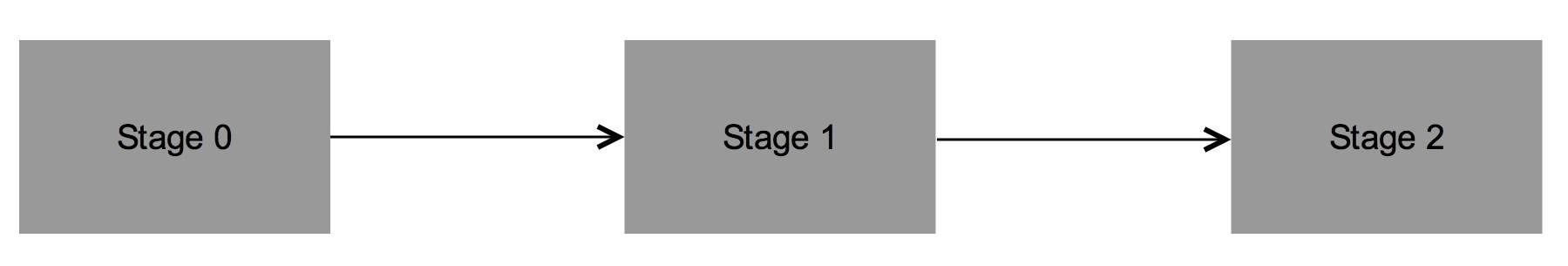## 5.2 Notation

Suppose that an individual may experience $$K$$ consecutive events at times $$T_1<T_2<\cdot\cdot\cdot<T_K=T$$, which are measured from the start of the follow-up.

Here different methods are proposed to estimate conditional survival probabilities such as $$P(T_2 > y \mid T_1 > x)$$ or $$P(T_2 > y \mid T_1 \leq x)$$, where $$T_1$$ and $$T_2$$ are ordered event times of two successive events.

The proposed methods are all based on the Kaplan-Meier estimator and the ideas behind the proposed estimators can also be used to estimate more general functions involving more than two successive event times. However, for ease of presentation and without loss of generality, we take $$K=2$$ in this section. The extension to $$K>2$$ is straightforward.Figure 5.2: 3-state progresive model.

Let $$(T_{1},T_{2})$$ be a pair of successive event times corresponding to two ordered (possibly consecutive) events measured from the start of the follow-up.

Let $$T=T_{2}$$ denote the total time and assume that both $$T_1$$ and $$T$$ are observed subject to a (univariate) random right-censoring variable $$C$$ assumed to be independent of $$(T_1,T)$$. Due to censoring, rather than $$(T_1,T)$$ we observe $$(\widetilde T_{1},\Delta_1,\widetilde T,\Delta_2)$$ where $$\widetilde T_{1}=\min (T_{1},C)$$, $$\Delta_{1}=I(T_{1}\leq C)$$, $$\widetilde T=\min (T,C)$$, $$\Delta_{2}=I(T\leq C)$$, where $$I(\cdot)$$ is the indicator function. Let $$(\widetilde T_{1i},\Delta_{1i},\widetilde T_i,\Delta_{2i})$$, $$1\leq i\leq n$$ be independent and identically distributed data with the same distribution as $$(\widetilde T_{1},\Delta_1,\widetilde T,\Delta_2)$$.